# 两点边值微分方程组多重正解的存在性Existence of Multiple Positive Solutions of Differential Equation System with Two-Point Boundary Value

DOI: 10.12677/AAM.2019.82026, PDF, HTML, XML, 下载: 473  浏览: 643  国家自然科学基金支持

Abstract: This paper deals with a class of two-point boundary value problems for differential equation system with parameters. Using fixed point index theory, existence of one positive solution and two positive solutions are obtained to this class of systems.

1. 引言

$\left\{\begin{array}{l}{u}^{\left(4\right)}\left(t\right)=f\left(t,v\right),\text{ }\text{ }\text{\hspace{0.17em}}\text{\hspace{0.17em}}\text{ }\forall \left(t,v\right)\in \left[0,1\right]×\left[0,+\infty \right),\\ -{v}^{″}\left(t\right)=g\left(t,u\right),\text{ }\text{ }\text{ }\forall \left(t,u\right)\in \left[0,1\right]×\left[0,+\infty \right),\\ u\left(0\right)=u\left(1\right)={u}^{″}\left(0\right)={u}^{″}\left(1\right)=0,\\ v\left(0\right)=v\left(1\right)=0.\end{array}$

$\left\{\begin{array}{l}{u}^{\left(4\right)}\left(t\right)=\lambda {h}_{1}\left(t\right)f\left(t,u\left(t\right),v\left(t\right)\right),\text{ }\forall t\in \left[0,1\right],\\ -{v}^{″}\left(t\right)=\mu {h}_{2}\left(t\right)g\left(t,u\left(t\right),v\left(t\right)\right),\text{ }\forall t\in \left[0,1\right],\\ u\left(0\right)=u\left(1\right)={u}^{″}\left(0\right)={u}^{″}\left(1\right)=0,\\ v\left(0\right)=v\left(1\right)=0.\end{array}$ (1)

(H1) $f,g:\left[0,1\right]×\left[0,+\infty \right)×\left[0,+\infty \right)\to \left[0,+\infty \right)$ ，且 $f,g$ 连续；

(H2) ${h}_{i}\in {L}^{1}\left[0,1\right]$${h}_{i}\left(t\right)\ge 0$ ，对 $\forall t\in \left[0,1\right]$${\int }_{\frac{1}{4}}^{\frac{3}{4}}{h}_{i}\left(t\right)\text{d}t>0$ ，其中 $i=1,2$

(H3) $\lambda ,\mu >0$ ，且为常数。

$\begin{array}{l}{f}_{0}=\underset{\left(u,v\right)\to {0}^{+}}{\mathrm{lim}}\text{inf}\frac{f\left(u,v\right)}{u+{v}^{2}},{f}^{0}=\underset{\left(u，v\right)\to {0}^{+}}{\mathrm{lim}}\text{sup}\frac{f\left(u,v\right)}{u+{v}^{2}},{f}_{\infty }=\underset{\left(u,v\right)\to \infty }{\mathrm{lim}}\text{inf}\frac{f\left(u,v\right)}{u+\sqrt{v}},{f}^{\infty }=\underset{\left(u,v\right)\to \infty }{\mathrm{lim}}\text{sup}\frac{f\left(u,v\right)}{u+\sqrt{v}}\\ {g}_{0}=\underset{\left(u,v\right)\to {0}^{+}}{\mathrm{lim}}\text{inf}\frac{g\left(u,v\right)}{u+{v}^{2}},{g}^{0}=\underset{\left(u,v\right)\to {0}^{+}}{\mathrm{lim}}\text{sup}\frac{g\left(u,v\right)}{u+{v}^{2}},{g}_{\infty }=\underset{\left(u,v\right)\to \infty }{\mathrm{lim}}\text{inf}\frac{g\left(u,v\right)}{u+\sqrt{v}},{g}^{\infty }=\underset{\left(u,v\right)\to \infty }{\mathrm{lim}}\text{sup}\frac{g\left(u,v\right)}{u+\sqrt{v}}\end{array}$

$K=\left\{\left(u,v\right)\in E|u\left(t\right)\ge 0,v\left(t\right)\ge 0,\underset{t\in \left[\frac{1}{4},\frac{3}{4}\right]}{\mathrm{min}}u\left(\text{t}\right)\ge \frac{1}{4}‖u‖,\underset{t\in \left[\frac{1}{4},\frac{3}{4}\right]}{\mathrm{min}}v\left(\text{t}\right)\ge \frac{1}{4}‖v‖,\forall t\in \left[0,1\right]\right\}$

2. 预备知识

1) $‖Ax‖\le ‖x‖,\forall x\in P\cap \partial {\Omega }_{1};‖Ax‖\ge ‖x‖,\forall x\in P\cap \partial {\Omega }_{2}$

2) $‖Ax‖\le ‖x‖,\forall x\in P\cap \partial {\Omega }_{2};‖Ax‖\ge ‖x‖,\forall x\in P\cap \partial {\Omega }_{1}$

1、当 $t,s\in \left[0,1\right]$ 时，有 $0\le G\left(t,s\right)\le G\left(t,t\right)$$0\le G\left(t,s\right)\le G\left(s,s\right)$

2、 $\frac{1}{4}G\left(x,s\right)\le G\left(t,s\right),\text{\hspace{0.17em}}\forall t\in \left[\frac{1}{4},\frac{3}{4}\right],\text{\hspace{0.17em}}\forall x,s\in \left[0,1\right]$

$\left\{\begin{array}{l}u\left(t\right)=\lambda {\int }_{0}^{1}{\int }_{0}^{1}G\left(t,s\right)G\left(\tau ,s\right){h}_{1}\left(\tau \right)f\left(u\left(\tau \right),v\left(\tau \right)\right)\text{d}\tau \text{d}s,\text{ }\text{\hspace{0.17em}}\text{\hspace{0.17em}}\text{\hspace{0.17em}}\forall t\in \left[0,1\right]\\ v\left(t\right)=\mu {\int }_{0}^{1}G\left(t,s\right){h}_{2}\left(s\right)g\left(u\left(s\right),v\left(s\right)\right)\text{d}s,\text{ }\text{ }\text{ }\text{ }\text{ }\text{\hspace{0.17em}}\text{\hspace{0.17em}}\text{\hspace{0.17em}}\text{\hspace{0.17em}}\forall t\in \left[0,1\right]\text{ }\end{array}$

$\Phi \left(u,v\right)=\lambda {\int }_{0}^{1}{\int }_{0}^{1}G\left(t,s\right)G\left(\tau ,s\right){h}_{1}\left(\tau \right)f\left(u\left(\tau \right),v\left(\tau \right)\right)\text{d}\tau \text{d}s,\text{ }\text{\hspace{0.17em}}\text{\hspace{0.17em}}\forall t\in \left[0,1\right]$

$\Psi \left(u,v\right)=\mu {\int }_{0}^{1}G\left(t,s\right){h}_{2}\left(s\right)g\left(u\left(s\right),v\left(s\right)\right)\text{d}s,\text{ }\text{\hspace{0.17em}}\text{\hspace{0.17em}}\text{\hspace{0.17em}}\text{\hspace{0.17em}}\forall t\in \left[0,1\right]$

${P}_{1}u\left(t\right)={\int }_{0}^{1}{\int }_{0}^{1}G\left(t,s\right)G\left(\tau ,s\right){h}_{1}\left(\tau \right)u\left(\tau \right)\text{d}\tau \text{d}s,\text{\hspace{0.17em}}\text{\hspace{0.17em}}\text{ }\text{ }\text{ }\text{ }\text{ }\text{ }\text{\hspace{0.17em}}\forall u\in C\left[0,1\right]$

${P}_{2}v\left(t\right)={\int }_{0}^{1}G\left(t,s\right){h}_{2}\left(s\right)v\left(s\right)\text{d}s,\text{\hspace{0.17em}}\text{\hspace{0.17em}}\text{\hspace{0.17em}}\forall v\in C\left[0,1\right]$ $P\left(u,v\right)\left(t\right)=\left({P}_{1}u\left(t\right),{P}_{2}v\left(t\right)\right),\text{ }\text{ }\text{ }\text{ }\text{ }\text{ }\text{ }\text{ }\text{ }\text{ }\text{ }\text{ }\text{ }\text{ }\text{ }\forall \left(u,v\right)\in E$

$H\left(u,v\right)\left(t\right)=\left(\lambda f\left(u\left(t\right),v\left(t\right)\right),\mu g\left(u\left(t\right),v\left(t\right)\right)\right),\text{ }\text{ }\text{ }\text{ }\text{ }\text{ }\forall \left(u,v\right)\in K$

$\begin{array}{l}T\left(u,v\right)=PH\left(u,v\right)=\left(\lambda {P}_{1}u\left(t\right),\mu {P}_{2}u\left(t\right)\right)\\ =\left(\lambda {\int }_{0}^{1}{\int }_{0}^{1}G\left(t,s\right)G\left(\tau ,s\right){h}_{1}\left(\tau \right)f\left(u\left(\tau \right),v\left(\tau \right)\right)\text{d}\tau \text{d}s,\mu {\int }_{0}^{1}G\left(t,s\right){h}_{2}\left(s\right)g\left(u\left(s\right),v\left(s\right)\right)\text{d}s\right)\end{array}$

$\begin{array}{c}T\left(u,v\right)\left(t\right)=\left(\lambda {\int }_{0}^{1}{\int }_{0}^{1}G\left(t,s\right)G\left(\tau ,s\right){h}_{1}\left(\tau \right)f\left(u\left(\tau \right),v\left(\tau \right)\right)\text{d}\tau \text{d}s,\mu {\int }_{0}^{1}G\left(t,s\right){h}_{2}\left(s\right)g\left(u\left(s\right),v\left(s\right)\right)\text{d}s\right)\\ \ge \frac{1}{4}\left(\lambda {\int }_{0}^{1}{\int }_{0}^{1}G\left(x,s\right)G\left(\tau ,s\right){h}_{1}\left(\tau \right)f\left(u\left(\tau \right),v\left(\tau \right)\right)\text{d}\tau \text{d}s,\mu {\int }_{0}^{1}G\left(x,s\right){h}_{2}\left(s\right)g\left(u\left(s\right),v\left(s\right)\right)\text{d}s\right)\\ =\frac{1}{4}T\left(u,v\right)\left(x\right)\end{array}$

$\underset{t\in \left[\frac{1}{4},\frac{3}{4}\right]}{\mathrm{min}}\Phi \left(u,v\right)\left(t\right)\ge \frac{1}{4}‖\Phi \left(u,v\right)‖\text{ }$$\underset{t\in \left[\frac{1}{4},\frac{3}{4}\right]}{\mathrm{min}}\Psi \left(u,v\right)\left(t\right)\ge \frac{1}{4}‖\Psi \left(u,v\right)‖$ ，所以 $T\left(K\right)\subset K$

${\phi }_{i}\in C\left[0,1\right]$ ，满足 ${\phi }_{i}\left(t\right)\ge 0,\text{\hspace{0.17em}}\forall t\in \left[0,1\right],\text{\hspace{0.17em}}{\phi }_{i}\left({t}_{0}\right)>0$${\phi }_{i}\left(t\right)=0,\text{\hspace{0.17em}}t\notin \left[\alpha ,\beta \right]$ ，则

$\begin{array}{c}{P}_{1}{\phi }_{1}\left(t\right)={\int }_{0}^{1}{\int }_{0}^{1}G\left(t,s\right)G\left(\tau ,s\right){h}_{1}\left(\tau \right){\phi }_{1}\left(\tau \right)\text{d}\tau \text{d}s\\ \ge {\int }_{\alpha }^{\beta }{\int }_{0}^{1}G\left(t,s\right)G\left(\tau ,s\right){h}_{1}\left(\tau \right){\phi }_{1}\left(\tau \right)\text{d}\tau \text{d}s>0,\text{ }\forall t\in \left[\alpha ,\beta \right]\end{array}$

${P}_{2}{\phi }_{2}\left(t\right)={\int }_{0}^{1}G\left(t,s\right){h}_{2}\left(s\right){\phi }_{2}\left(s\right)\text{d}s>{\int }_{\alpha }^{\beta }G\left(t,s\right){h}_{2}\left(s\right){\phi }_{2}\left(s\right)\text{d}s>0,\text{ }\text{ }\text{ }\text{ }\text{ }\text{ }\text{ }\text{ }\text{ }\text{ }\text{ }\text{ }\text{ }\forall t\in \left[\alpha ,\beta \right]$

${\lambda }_{1}$ 为线性边值问题 $\left\{\begin{array}{l}{u}^{\left(4\right)}\left(t\right)=\lambda {h}_{1}\left(t\right)u\left(t\right),\text{ }\forall t\in \left(0,1\right)\\ u\left(0\right)=u\left(1\right)={u}^{″}\left(0\right)={u}^{″}\left(1\right)=0\end{array}$ 的第一个特征值， ${\mu }_{1}$ 为线性边值问题 $\left\{\begin{array}{l}-{v}^{″}\left(t\right)=\mu {h}_{2}\left(t\right)v\left(t\right),\text{\hspace{0.17em}}\text{\hspace{0.17em}}\forall t\in \left(0,1\right)\\ v\left(0\right)=v\left(1\right)=0\end{array}$ 的第一个特征值，则 ${\lambda }_{1},{\mu }_{1}>0$ 。从而 ${P}_{1}$ 的谱半径 ${r}_{1}=\frac{1}{{\lambda }_{1}}>0$${P}_{2}$ 的谱半径 ${r}_{2}=\frac{1}{{\mu }_{1}}>0$ 。假设它们相对应的特征函数分别为 ${\phi }_{1},{\phi }_{2}$ 。则满足 ${\int }_{0}^{1}{h}_{i}\left(t\right){\phi }_{i}\left(t\right)>0$ ，否则如果 ${\int }_{0}^{1}{h}_{i}\left(t\right){\phi }_{i}\left(t\right)=0$ ，则

${r}_{1}{\phi }_{1}\left(t\right)={P}_{1}{\phi }_{1}\left(t\right)={\int }_{0}^{1}{\int }_{0}^{1}G\left(t,s\right)G\left(\tau ,s\right){h}_{1}\left(\tau \right){\phi }_{1}\left(\tau \right)\text{d}\tau \text{d}s\le {M}^{2}{\int }_{0}^{1}{h}_{1}\left(\tau \right){\phi }_{1}\left(\tau \right)\text{d}\tau =0$

${r}_{2}{\phi }_{2}\left(t\right)={P}_{2}{\phi }_{2}\left(t\right)={\int }_{0}^{1}G\left(t,s\right){h}_{2}\left(s\right){\phi }_{2}\left(s\right)\text{d}s

$z\left(u,v\right)={\int }_{0}^{1}\left[{\phi }_{1}\left(t\right){h}_{1}\left(t\right)u\left(t\right)+{\phi }_{2}\left(t\right){h}_{2}\left(t\right)v\left(t\right)\right]\text{d}t,\text{ }\forall \left(u,v\right)\in E$

$z\left(u,v\right)\ge \frac{1}{4}\left(‖u‖{m}_{1}+‖v‖{m}_{2}\right)\ge m‖\left(u,v\right)‖$ (2)

$\begin{array}{c}z\left(P\left(u,v\right)\right)={\int }_{0}^{1}\left[{\phi }_{1}\left(t\right){h}_{1}\left(t\right){\int }_{0}^{1}{\int }_{0}^{1}G\left(t,s\right)G\left(\tau ,s\right){h}_{1}\left(\tau \right)u\left(\tau \right)\text{d}\tau \text{d}s+{\phi }_{2}\left(t\right){h}_{2}\left(t\right){\int }_{0}^{1}G\left(t,s\right){h}_{2}\left(s\right)v\left(s\right)\text{d}s\right]\text{d}t\\ ={\int }_{0}^{1}\left[{\phi }_{1}\left(t\right){h}_{1}\left(t\right){\int }_{0}^{1}{\int }_{0}^{1}G\left(t,s\right)G\left(\tau ,s\right){h}_{1}\left(\tau \right)u\left(\tau \right)\text{d}\tau \text{d}s+{\phi }_{2}\left(t\right){h}_{2}\left(t\right){\int }_{0}^{1}G\left(t,\tau \right){h}_{2}\left(\tau \right)v\left(\tau \right)\text{d}\tau \right]\text{d}t\\ ={\int }_{0}^{1}\left[{h}_{1}\left(\tau \right)u\left(\tau \right){\int }_{0}^{1}{\int }_{0}^{1}G\left(\tau ,s\right)G\left(s,t\right){h}_{1}\left(t\right){\phi }_{1}\left(t\right)\text{d}t\text{d}s+{h}_{2}\left(\tau \right)v\left(\tau \right){\int }_{0}^{1}G\left(\tau ,t\right){h}_{2}\left(t\right){\phi }_{2}\left(t\right)\text{d}t\right]\text{d}\tau \end{array}$

$z\left(P\left(u,v\right)\right)={\int }_{0}^{1}\left[{h}_{1}\left(\tau \right)u\left(\tau \right){r}_{1}{\phi }_{1}\left(\tau \right)+{h}_{2}\left(\tau \right)v\left(\tau \right){r}_{2}{\phi }_{2}\left(\tau \right)\right]\text{d}\tau$ (3)

3. 主要结果

1) 由 $\lambda {f}^{0}<{\lambda }_{1},\mu {g}^{0}<{\mu }_{1}$ 可得 $\exists \delta \in \left(0,1\right),d>0$ 且充分小，使得当 $\left(u,v\right)\in K,‖\left(u,v\right)‖\le d$ 时，对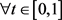，恒有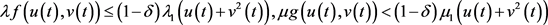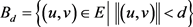，假设存在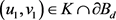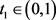满足，则由(3)式得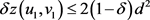，由(2)式得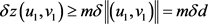，即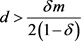，这与d充分小矛盾。根据不动点指数的同伦不变性得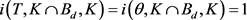2) 由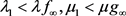可得，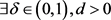，使得当时，有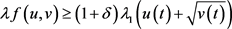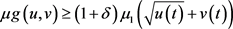。又当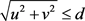时，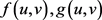有界，故存在常数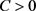，使得当时，对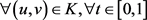，恒有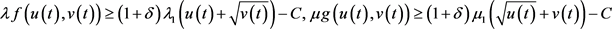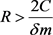，令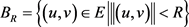，假设存在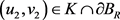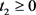满足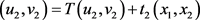，其中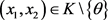，则由(3)可得1) 由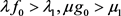可得，使得当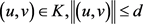时，对，有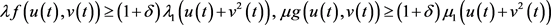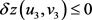，这与(2)式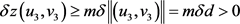矛盾，从而假设不成立。由不动点指数的缺方向性知，当d充分小时，有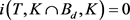2) 又由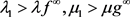可得，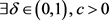，使得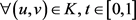时，有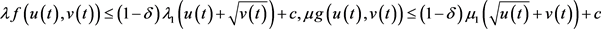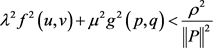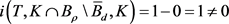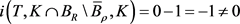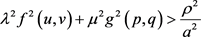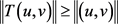，故由锥压缩定理知，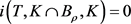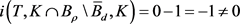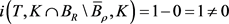郭大钧. 非线性泛函分析[M]. 第3版. 济南: 山东科技出版社, 1985: 243-244.  姚庆六, 白占兵. 的边值问题的正解存在性[J]. 数学年刊: 中文版, 1999(5): 575-578.  席莉静. 一类算子方程的多重正解及对奇异边值问题的应用[J]. 苏州科技大学学报(自然科学版), 2004, 21(3): 26-30.  陈丽珍, 李刚. 四阶非线性微分方程组正解的存在性[J]. 扬州大学学报(自然科学版), 2008, 11(4): 25-28.  王全义, 邹黄辉. 一类四阶奇异非线性积分边值问题正解的存在性[J]. 华侨大学学报, 2014, 35(1): 83-87.  赵微. 一类四阶微分方程m点边值问题的正解[J]. 四川师范大学学报(自然科学版), 2017, 40(6): 791-796.  Jankowski, T. (2010) Positive Solutions for Fourth-Order Differential Equations with Deviating Argu-ments and Integral Boundary Conditions. Nonlinear Analysis, 73, 1289-1299. https://doi.org/10.1016/j.na.2010.04.055  马田田, 赵增勤. 非线性微分方程组边值问题多个正解的存在性[J]. 工程数学学报, 2009, 26(6): 1050-1056.  周韶林, 吴红萍, 韩晓玲. 一类四阶三点边值问题正解的存在性[J]. 四川大学学报(自然科学版), 2014, 51(1): 11-15.